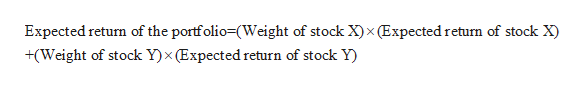# You have \$24,000 to invest in a stock portfolio. Your choices are Stock X with an expected return of 13 percent and Stock Y with an expected return of 12 percent. If your goal is to create a portfolio with an expected return of 12.65 percent, how much money will you invest in Stock X and Stock Y? (Do not round intermediate calculations and round your answers to the nearest whole number, e.g., 32.)

Question
66 views

You have \$24,000 to invest in a stock portfolio. Your choices are Stock X with an expected return of 13 percent and Stock Y with an expected return of 12 percent. If your goal is to create a portfolio with an expected return of 12.65 percent, how much money will you invest in Stock X and Stock Y? (Do not round intermediate calculations and round your answers to the nearest whole number, e.g., 32.)

check_circle

Step 1

Given that the total investment amount is \$24,000, expected return on stock X is 13% and expected return on stock Y is 12%, expected portfolio return is 12.65%, we need to determine the amounts we need to invest in Stock X and Stock Y.

Step 2

To determine the investment amounts, first we need to determine the weights of individual stocks in the portfolio.
Suppose the weight of stock X be X, then the weight of stock Y will be (1-X)

Step 3help_outlineImage TranscriptioncloseExpected return of the portfolio=(Weight of stock X)x (Expected return of stock X) +(Weight of stock Y)x (Expected return of stock Y) fullscreen

### Want to see the full answer?

See Solution

#### Want to see this answer and more?

Solutions are written by subject experts who are available 24/7. Questions are typically answered within 1 hour.*

See Solution
*Response times may vary by subject and question.
Tagged in